# GSEB Solutions Class 12 Statistics Part 1 Chapter 1 Index Number Ex 1.1

Gujarat Board Statistics Class 12 GSEB Solutions Part 1 Chapter 1 Index Number Ex 1.1 Textbook Exercise Questions and Answers.

## Gujarat Board Textbook Solutions Class 12 Statistics Part 1 Chapter 1 Index Number Ex 1.1

Question 1.
The data about average daily wage of a group of workers employed in a factory in a city during the year 2008 to 2015 are as follows. Find the index number by (1) Fixed base method (taking base year 2008), (2) Chain base method and (3) Fixed base method by taking average of average daily wages of the years 2011 to 2013 as the wage for the base year.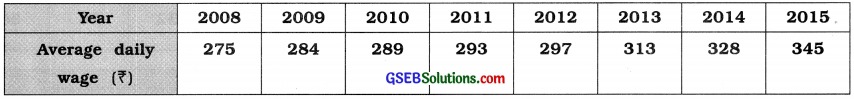Question 2.
From the following data about the retail prices of sugar in a city, find the index numbers of price of sugar by (1) Fixed base method with year 2008 as base year, (2) Chain base method and (3) Taking the average price of sugar for the year 2009 and 2010 as the base year price.Question 3.
The following data are obtained about the annual average prices of wheat, rice and sugar in the wholesale market of a city. Find the general index number for three items by fixed base method with base year 2011 and by chain base method.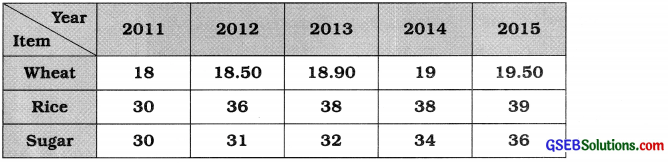General index number of price by Fixed Base method: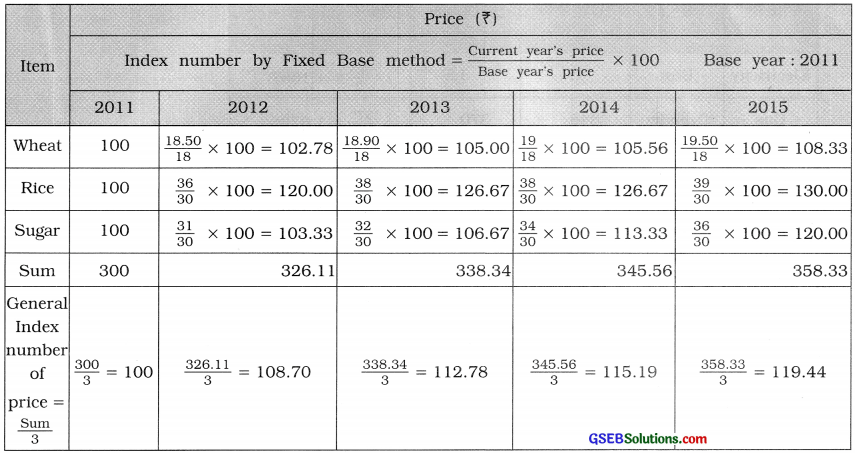General index number of price by Chain Base method: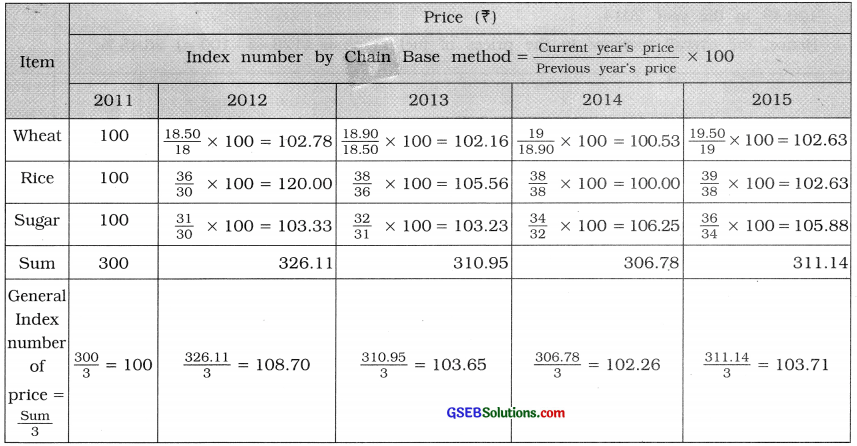Question 4.
The prices of five fuel related items in the years 2012 and 2014 are as follows. Calculate the general index number for five fuel items by taking the year 2012 as the base year and state the overall increase in the prices of fuel items.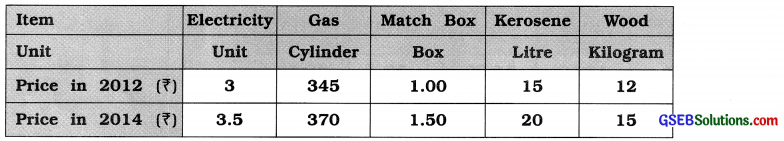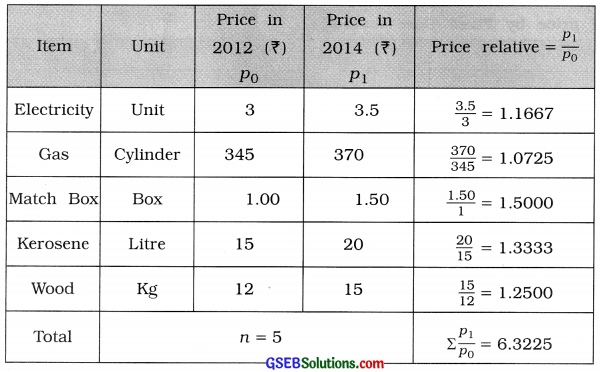= $$\frac{\sum\left(\frac{p_{1}}{p_{0}}\right)}{n}$$ × 100
= $$\frac{6.3225}{5}$$ × 100